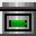Search
Software
Options for InvestaCalc Investment Calculator
InvestaCalc Investment CalculatorInvestaCalc 4.0 is a investment and loan amortization calculator. There are a total of 18 financial functions which include the ability to compute the future value of periodic and lump-sum investments, beginning value of periodic withdrawals, and generate simple amortization tables.

There are several more financial and investment functions included. Calculate the Effective Annual Rate, the Future Value of Uneven Cash Flow, Probability Distributions, and even the ability to calculate the CAPM Rate of Return.

InvestaCalc Functions:

* Lump-Sum Investments
- Future Value
- Beginning Value
- Number of Periods
- Rate of Return
- Periodic Investments

* Future Value
- Beginning Value
- Number of Periods
- Rate of Return
- Periodic Withdrawals (Annuities)

* Beginning Value
- Periodic Withdrawal
- Number of Periods
- Rate of Return
- Effective Annual Rate

* Future Value of Uneven Cash Flow
* Probability Distribution
* Standard Deviation
* Capital Asset Pricing Model - CAPM
* Amortization table generation